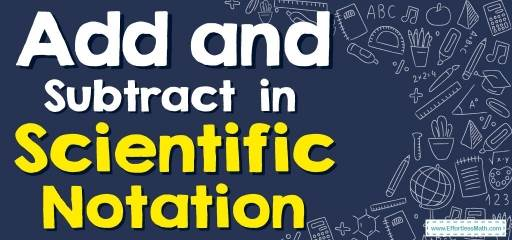# How to Add and Subtract in Scientific Notations? (+FREE Worksheet!)

Scientific notation is one of the most common methods in mathematics for displaying very large and very small numbers that make calculations with those numbers easier. This article teaches you how to add and subtract in Scientific Notations using a few simple steps.Scientific notation is one of the most common methods in mathematics for displaying very large and very small numbers that make calculations with those numbers easier. With a scientific notation, each number can be written as a product of two numbers.

To add or subtract numbers in scientific notion, we need to have the same power of the base (number $$10$$), and only decimal parts are added or subtracted.

## Step by step guide to Add and Subtract Scientific Notations

Adding and subtracting numbers in scientific notion:

• Step 1: Adjust the powers in the numbers so that they have the same power. (It is easier to adjust the smaller power to equal the larger one)
• Step 2: Add or subtract the numbers (only decimal parts).
• Step 3: Convert the answer to scientific notation if needed.

### Addition and Subtraction in Scientific Notation– Example 1:

Write the answer in scientific notation. $$11\times 10^7 -\ 4.4\times 10^7=$$

Solution:

Since two numbers have the same power, factor $$10^7$$ out: $$(11 -\ 4.4 ) \times 10^7 = 6.6\times 10^7$$

### Addition and Subtraction in Scientific Notation– Example 2:

Write the answer in scientific notation. $$9.7\times 10^4 -\ 33\times 10^3=$$

Solution:

Convert the second number to have the same power of $$10$$: $$33\times 10^3=3.3\times 10^4$$.

Now, two numbers have the same power of $$10$$. Subtract: $$9.7\times 10^4 -\ 3.3\times 10^4 = (9.7 -\ 3.3 ) \times 10^4 = 6.4\times 10^4$$

### Addition and Subtraction in Scientific Notation– Example 3:

Write the answer in scientific notation. $$3.5\times 10^6 +\ 4.7\times 10^6=$$

Solution:

Since two numbers have the same power, factor $$10^6$$ out: $$(3.5 +\ 4.7 ) \times 10^6 = 8.2\times 10^6$$

### Addition and Subtraction in Scientific Notation– Example 4:

Write the answer in scientific notation. $$2.6\times 10^8 +\ 4.4\times 10^7=$$

Solution:

Convert the second number to have the same power of $$10$$: $$4.4\times 10^7=0.44\times 10^8$$.

Now, two numbers have the same power of $$10$$. Add: $$2.6\times 10^8 +\ 0.44\times 10^8 = (2.6 +\ 0.44 ) \times 10^8 = 3.04\times 10^8$$

## Exercises for Adding and Subtracting Scientific Notations

### Write the answer in scientific notation.

1. $$\color{blue}{5.1\times 10^5 +\ 3.9\times 10^5=}$$
2. $$\color{blue}{8.9\times 10^7 -\ 6.9\times 10^7=}$$
3. $$\color{blue}{1.2\times 10^4 +\ 3\times 10^3=}$$
4. $$\color{blue}{5.3\times 10^6 -\ 2.2\times 10^5=}$$
5. $$\color{blue}{1.6\times 10^9 +\ 4.8\times 10^9=}$$
6. $$\color{blue}{9.8\times 10^3 -\ 6.1\times 10^3=}$$
1. $$\color{blue}{9\times 10^5}$$
2. $$\color{blue}{2\times 10^7}$$
3. $$\color{blue}{1.5\times 10^4}$$
4. $$\color{blue}{5.08\times 10^6}$$
5. $$\color{blue}{6.4\times 10^9}$$
6. $$\color{blue}{3.7\times 10^3}$$

### What people say about "How to Add and Subtract in Scientific Notations? (+FREE Worksheet!) - Effortless Math: We Help Students Learn to LOVE Mathematics"?

No one replied yet.

X
30% OFF

Limited time only!

Save Over 30%

SAVE $5 It was$16.99 now it is \$11.99